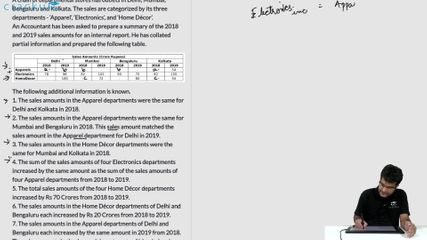### CAT 2020 Question Paper (Slot 2) Question 30

Instructions

A chain of departmental stores has outlets in Delhi, Mumbai, Bengaluru and Kolkata. The sales are categorized by its three departments - ‘Apparel’, ‘Electronics’, and ‘Home Décor’. An Accountant has been asked to prepare a summary of the 2018 and 2019 sales amounts for an internal report. He has collated partial information and prepared the following table.

The following additional information is known.
1. The sales amounts in the Apparel departments were the same for Delhi and Kolkata in 2018.
2. The sales amounts in the Apparel departments were the same for Mumbai and Bengaluru in 2018. This sales amount matched the sales amount in the Apparel department for Delhi in 2019.
3. The sales amounts in the Home Décor departments were the same for Mumbai and Kolkata in 2018.
4. The sum of the sales amounts of four Electronics departments increased by the same amount as the sum of the sales amounts of four Apparel departments from 2018 to 2019.
5. The total sales amounts of the four Home Décor departments increased by Rs 70 Crores from 2018 to 2019.
6. The sales amounts in the Home Décor departments of Delhi and Bengaluru each increased by Rs 20 Crores from 2018 to 2019.
7. The sales amounts in the Apparel departments of Delhi and Bengaluru each increased by the same amount in 2019 from 2018. The sales amounts in the Apparel departments of Mumbai and Kolkata also each increased by the same amount in 2019 from 2018.
8. The sales amounts in the Apparel departments of Delhi, Kolkata and Bengaluru in 2019 followed an Arithmetic Progression.

Question 30

# What was the total sales amount, in Crore Rupees, in 2019 for the chain of departmental stores?

Solution

From 1, Let sales amounts in the Apparel departments for Delhi and Kolkata in 2018 be a.

From 2, Let sales amounts in the Apparel departments for Mumbai and Bengaluru in 2018 and for Delhi be b.

From 3, let the sales amounts in the Home Décor departments for Mumbai and Kolkata in 2018 be c and that of Delhi and Bengaluru be s and t respectively.

Let the Let sales amounts in the Apparel departments for Mumbai and Bengaluru in 2019 be m and n respectively.

The table looks like:

From 6, s=80 and t=60.
From 5, s+c+t+c+70=306 => 2c=96 => c=48.

From 4, (b-a)+(m-b)+(n-b)+(54-a)=(20)+20-20+20 => 54+m+n-2a-b=40=> 2a+b=14+m+n => 2b=28+2m+2n-4a...(i)

From 7, b-a=n-b => 2b=a+n...(ii) and m-b=54-a=> a+m=54+b=>2b=2a+2m-108...(iii)

From 8, 108=b+n=>2b=216-2n...(iv)

from (i) & (iii), 28+2m+2n-4a=2a+2m-108 => 6a=136+2n => 3a=68+n...(v)

from (ii) & (iv), 216-2n=a+n => a+3n=216=> 3a=648-9n...(vi)

from (v) & (vi), 648-9n=68+n => n=58 => a=42 => b=50 => m=62.

The final table looks like this

.'. total sales amount, in Crore Rupees, in 2019 for the chain of departmental stores =900 cr.

### View Video Solution